Maths-
General
Easy

Question

# Find the Total surface area if the given dimensions are 6 cm,4cm, and 5 cm.

## 164 cm2120 cm2148 cm2134 cm2Hint:

## The correct answer is: 148 cm2

### We will calculate the total surface area of a cuboid by using the following formula: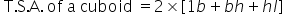The dimensions of the given cuboid:Length, l = 4 cmBreadth, b = 5 cmHeight, h = 6 cmBy using the above formula of the total surface area of the cuboid, we getThe total surface area of the given cuboid is,=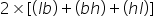=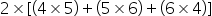=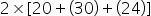=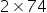= 148 cm2Thus, the total surface area of a cuboid of dimensions 6 cm, 4 cm & 5 cm is 148 cm².The correct option is C)148 cm².Note:- In some examples it may be given the surface area and any two dimensions , then we have to adjust the formula such a that we will be able to find out the required value . For eg- If area , length and breadth is given the formula for height becomes#### With Turito Foundation.#### Get an Expert Advice From Turito.